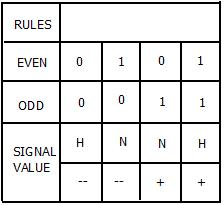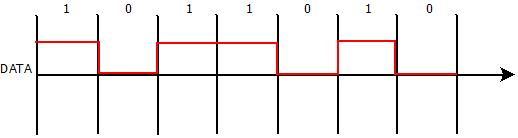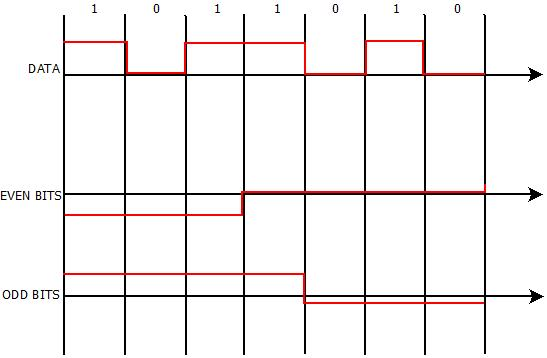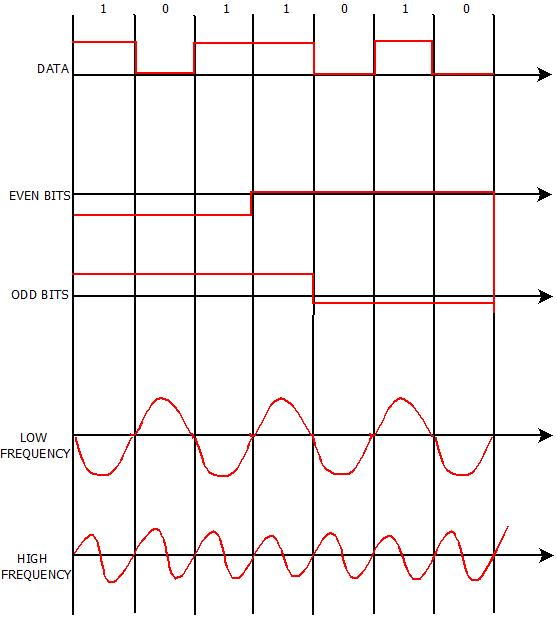# MINIMUM SHIFT KEY MOBILE COMPUTING

Minimum Shift Key (MSK) | Mobile Computing Tutorial | Minigranth

# Minimum Shift Key/Advance Frequency Shift Key : Introduction

• Minimum Shift Key is another type of digital modulation technique which can be used to convert digital signal into analog signals.
• Minimum Shift Key is much more effective and can be implemented for almost every stream of bits much easily than Phase Shift Key, Frequency Shift Key and Amplitude Shift Key.
• The concept of Minimum Shift Key is based upon the positioning of bits i.e. even bits and odd bits for the given bit stream and the bit positioning frequency generating table.
• MSK is widely used because of its ability and flexibility to handle “One(1)” and “Zero(0)” transition of binary bits.

### Minimum Shift Key : Working

• In MSK, bits are separated in even and odd bits and duration of each bit is doubled.
• Then the frequency f1 and f2 are chosen in which f1 determines/denotes low frequency and f2 denotes high frequency.
• Depending upon the bit values, even or odd, original or inverted signal is chosen from frequency generating table.
• Higher frequency curve takes a complete wave from 0 to π and low frequency curve takes a wave 0 to π/2 within same interval of time.

For Example : Consider a bit stream : 1011010. We need to find the MSK curve for this bit stream.

Rules:MSK Rules

Step-1 : Draw the curve as per the amplitude. If the bit is zero, it have amplitude while bit is zero, it does not.Minimum Shift Key : Step-1

Step-2 : Starting with the odd bit, if it is one draw the curve above the x-axis twice as long as the original one. If the bit is zero draw the curve below the x-axis twice as long as the original one.Minimum Shift Key : Step-2

Step-3 :Draw the curve for high and low frequency as shown below. It would remain same for any problem.Minimum Shift Key : Step-3

Step-4 : Draw the final curve as taking the frequency generating table. The blue colored curve represents the final obtained MSK curve.Minimum Shift Key : Step-4